IMO Shortlist 2011 problem C6

Kvaliteta:
Avg: 3,0
Težina:
Avg: 8,0
Let$n$ be a positive integer, and let$W = \ldots x_{-1}x_0x_1x_2 \ldots$ be an infinite periodic word, consisting of just letters$a$ and/or$b$. Suppose that the minimal period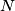$N$ of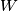$W$ is greater than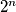$2^n$.

A finite nonempty word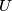$U$ is said to appear in$W$ if there exist indices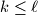$k \leq \ell$ such that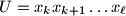$U=x_k x_{k+1} \ldots x_{\ell}$. A finite word$U$ is called ubiquitous if the four words$Ua$,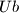$Ub$,$aU$, and$bU$ all appear in$W$. Prove that there are at least$n$ ubiquitous finite nonempty words.

Proposed by Grigory Chelnokov, Russia
Izvor: Međunarodna matematička olimpijada, shortlist 2011